# What Is ValueError in Python and How to Fix It?One of the trickier parts of designing and writing code remains to be exception handling. Poor exception handling causes a number of issues, including:

• Poorly designed code that is challenging to test, understand and refactor.
• It is challenging to identify the primary cause of errors.
• Poor monitoring and understanding.

These issues not only make it challenging to restructure and add new features to the code but also make it difficult to manage the application in real-world settings. One of those exceptions is ValueError and we will discuss in this article what ValueError is and how to fix it. Let us go through everything about ValueError in Python.

## What is ValueError in Python?

An exception is referred to as occurring during the execution of any script. In Python, exception handling is done with the try-except block. Python has a large number of built-in exceptions to deal with typical problems, like IndexError, KeyError, NameError, TypeError, ValueError, etc. Python's ValueError occurs when a function is called with the proper argument type but with the wrong value. This kind of mistake frequently occurs during mathematical calculations, when a ValueError Python arises. We will discuss the process to handle it in Python.

When a user calls a function with an invalid value but a valid argument, Python raises ValueError. Even though the value is the correct argument, it typically happens in mathematical processes that call for a specific kind of value. When an object is given the incorrect value, the Python ValueError is raised. This may occur if the value is incorrect for the intended operation or if the value is missing altogether. A ValueError is raised, for instance, if a negative integer is supplied to a square root operation.

ValueError example 1:

Code

``````a=10
b=int('valueerror')
print(b)
``````

Output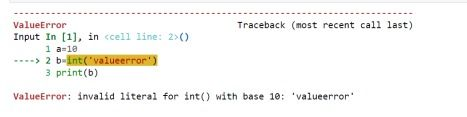Explanation

The output shown after running the aforementioned script is as follows. The output indicates that the ValueError occurred at line 2, where the string "valueerror" was converted using the int() function.

ValueError example 2:

Here is an illustration of a Python ValueError that occurs while attempting to square the root a negative number:

Code

``````import math
a=-10
print(math.sqrt(a))
``````

Output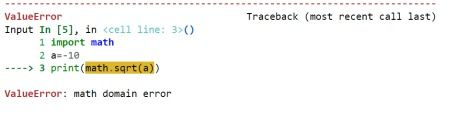Explanation

The math.sqrt() function is given a negative integer in the example above. Running the aforementioned code results in a ValueError since the function expects a positive number.

Before moving on to the topic of how to fix it let us see one exciting topic in ValueError.

## ValueError - Unpacking

Retrieving values from a list and assigning them to a list of variables is referred to as unpacking. When the number of variables and values don't match, this error occurs. The inequality prevents Python from knowing which values to assign to which variables. This results in the ValueError: too many values to unpack the problem.

Now let us discuss two problems of the exception mentioned above and how to solve them:

First problem: Occurs when list unpacking

Imagine you have a list of fruits and you want to give a few variables the values from the list.

This procedure can be imitated below:

``````>>fruits = ['Apple', 'Mango', 'orange']
>>fruits_1, fruits_2 = fruits
``````

Output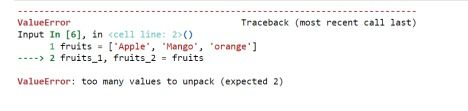Because there are three values in the list but we only assign them to two variables, we are receiving this traceback.

Python is therefore unable to determine which fruit we prefer - Apple, Mango, or Orange. We can resolve this quickly:

``````>>fruits = ['Apple', 'Mango', 'Orange']
>>fruits_1, fruits_2,fruits_3 = fruits
``````

fruits_3 makes this section of code function properly because we now have an equal number of variables and list values.

Apple, Mango, and Orange are now assigned to fruits_1, fruits_2, and fruits_ 3, respectively, via this change's communication to Python.

Second Problem: Unpacking when a return statement is used in a function. Consider that we wish to create a function that computes the values of variables - variable 1 and variable 2, then returns the results for usage elsewhere in our program.

Here is a function that specifically provides us with the sum, and difference of two variables:

Code

``````def computes(x, y):
sum = x + y
diff= x - y
mul=x*y
return sum, diff, mul

result_1, result_2,result_3 = computes(7, 5)
``````

Output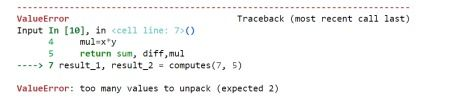The function returns three variables, but we only requested two, hence this error happens.

Python doesn't know which two variables we're looking for, therefore the value error informs us that we've made a mistake rather than presuming and giving us simply two values (which can destroy our program later if they're the incorrect values).

You have a few choices for how to effectively capture the function's output. The below improvement includes methods to avoid this problem.

``````def computes(x, y):
sum = x + y
diff= x - y
mul=x*y
return sum, diff,mul
result_1, result_2,result_3 = computes(7, 5)
print(f"Option 1: sum={result_1}, diff={result_2}, mul={result_3}")
``````

The program can now run without errors because Python can now directly link the return of sum, product, and difference to result 1, result 2, and result 3, respectively.

Another option for improvement to the above program can also include just underscore instead of result_3 and return the output with any variables assigned.

In the output, while printing you can also use just results instead of separately declaring result_1,result_2, and result_3 and extracting the result like you will get the element in the array.

## Most common uses of ValueError exception

Example-1: Handle the ValueError for incorrect data

The script below should be placed in a Python file to collect the user's age value. The try block will throw the ValueError exception and output the custom error message if the user enters a non-numeric value for the age field. If the user's actual age is obtained, the message will be printed using that age value.

Code

``````try:
age = int(input("Enter your age: "))

if age >= 18 :
print("You are eligible to vote.")
else:
print("You are not eligible to vote.")

except ValueError:
``````

Output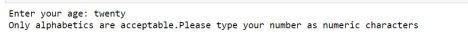As the above problem is a basic program for user to check if he is eligible to vote or not. So there is a possibility for the user to type non-numeric values as you can see in the above screenshot.

Example-2: Usage of the ValueError Python inside and outside of the function

The following Python program will demonstrate how ValueError is used both inside and outside of the function. Here, it has been defined to use the sign() function to determine whether an integer is positive or negative. When an invalid argument value is supplied to the process, the function will show the ValueError. The ValueError provided by the function will be caught by the try-except block, which will then provide an error message.

Code

``````def sign(n):
try:
value = int(n)
#Checking the number is positive or negative
if value> 0:
print("The number is positive")
else:
print("The number is negative")
except ValueError:

print("Error occurred inside the function: ", "Please Correct") raise
try:
#Take the input from the user
number = input("Enter a number a value: ")
#Calling the function
sign(number)
except ValueError:
print("Error occurred outside the function: ", "Please correct")
``````

Output: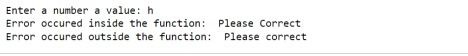The above problems explains whether the value entered by the user is a positive or negative number. If there is any input other than a number, it will show an exception inside and outside of the function.

## Other uses of ValueError

The following Python script will open a file for reading and print the contents of the file. Create a Python file. An IOError will be shown if the filename used in the script is not reachable, and a ValueError will be generated if the file includes any alphabetic characters.

Example 2: Command line argument

The following Python script will take a number from the value of the command-line argument. If a numeric value is provided in the command-line argument, the specific message will be written; if not, a ValueError will be issued and an error message will be displayed.

## How ValueError Python is fixed

A try-except block can be used in Python programs to fix ValueError. The try block should contain the lines of code that can show the ValueError, and the except block should have the code to catch and handle the problem.

Code

``````import math

try:
math.sqrt(-10)
except ValueError:
print('Positive number is required for square root operation. So please change the number')
``````

Output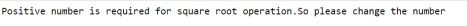The try-except block is used in this case to check for the ValueError. The except block in the code above detects the ValueError and handles it, resulting in the output shown above.

## Conclusion

It is always for a software developer to handle any exceptions that occurs in a program. To be a successful developer it is always important to be a good tester, debugger, and compiler. So we have seen what ValueError is in Python and how to fix it using try and except.

The value in Python is the data that is stored inside a particular item. A ValueError in Python indicates that there is a problem with the program typed that you tried to assign the incentive to. This should not be confused with Python types. Therefore, when a proper and valid argument is received, Python ValueError occurs; however, it is not really worth it. Additionally, the situation shouldn't be described by a more precise exception, like IndexError.

## Author

•### S.Harihara Sudhan

Harihara Sudhan is an upcoming data scientist in Japan and actively participates in writing technical blogs. He is an open-source contributor who has contributed technical writing to many events. He has developed many contents in machine learning, Information technology.

In Python, exceptions are caught and dealt with using the try and except commands for the statements with exception-raising potential. The code that will be prone to exceptions is written inside the try block and the exception message should be written inside except block.

While supplying arguments with the incorrect value (such as a number outside of expected boundaries) should result in a ValueError, passing arguments of the incorrect type (such as passing a list when an int is intended) should result in a TypeError.

An Exception may represent conditions that a program should strive to capture, but an Error may represent serious issues that would be unreasonable for it to catch.### Press

What's up with Turing? Get the latest news about us here.### Blog